# How is refraction used in everyday life

## Refraction / refraction of light

We deal with the refraction of light in this article. Summary of the content:

• First one Explanationwhat is meant by the refraction of light and what formula to use.
• Examples with numbers to clarify the relationships.
• tasks / Exercises so that you can train yourself.
• A Videowhich explains the refraction of light.
• A Question and answer area with typical questions about the refraction of light.

We'll look at the refraction of light in a moment. If you don't know what to do with the term light, you should first take a look at the article Optics Basics. The relevant basics are explained there. Everyone else can continue with this article right away.

### Refraction explanation

How can you imagine the refraction of light? Well, go to the swimming pool and stand at the edge of a pool. And now look into the pool. Then you should notice that what you see in the water looks kind of strange. It looks like you have a "kink" in the optics.

So let's take a swimming pool with water (blue) and of course there is air above it:And there is now a ray of light on it from above, marked in red: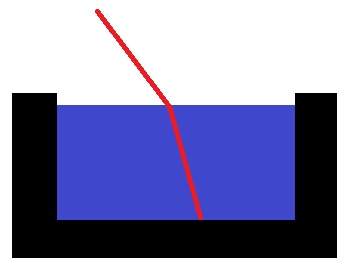As you can see, the light beam makes a kink as soon as it hits the surface of the water. This is also not uncommon. And you can even calculate this.

Refraction formula:

How can you calculate the refraction of light? For this we look at the formula for the law of refraction. The following applies: Light passes from one translucent medium to another translucent medium at an interface. To the following graphic ...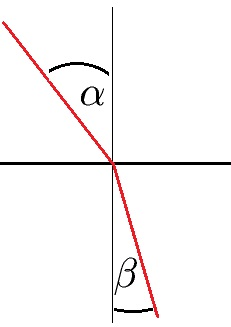.. now these formulas apply: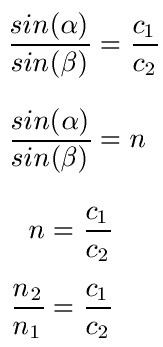Where:

• "α" is the angle of incidence
• "β" is the angle of reflection / angle of refraction
• "c1"is the speed of light in the first substance
• "c2"is the speed of light in the second substance
• "n" is the refractive index
• "n1"is the refractive index of the first substance
• "n2"is the refractive index of the second substance
Display:

### Examples of refraction of light

Let's look at examples of how light is refracted.

example 1:

The speed of light in air is 300,000 kilometers per second and the speed of light in water is 225,000 kilometers per second. What is the refractive index n?

Solution: We can insert the information directly and thus calculate n = 1.33.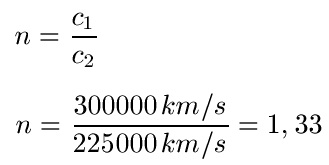Example 2:

Light hits the surface of the water at an angle of 45 degrees. The refractive index is n = 1.3. Calculate the angle of reflection.

Solution: We need beta at the end, so we first convert the formula to sinus beta. Then we insert the 45 degrees and the 1.3 here. We calculate the right-hand side of the equation with the pocket calculator, which must be set to DEG. To get the sine away before beta we need to use arcsin on the calculator. Arcsin is sometimes also sin on the calculator-1. This gives us an angle of reflection of almost 33 degrees.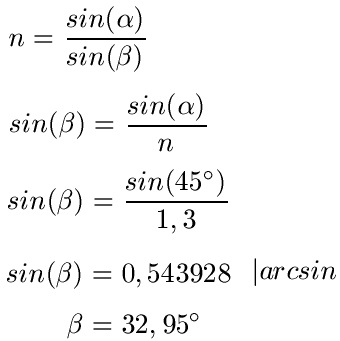Show:

### Refraction of Light Videos

This video is about the refraction of light. This will first be explained using the example of fishing in water, because this is where the phenomenon of light refraction comes into play. The terms solder as well as optically denser medium and optically thinner medium are explained and how the light is refracted on the water surface. Corresponding angle designations are also introduced. In the second example, an optically denser medium is changed to the optically thinner medium, i.e. a light source is in the water and radiates out of the pool. As a third example, we have a light beam that hits a glass plate and there is a parallel offset. I found this video on Youtube.com.

Next video »